# Minkowski spacetime facts for kids

Kids Encyclopedia Facts

In special relativity, the Minkowski spacetime is a four-dimensional manifold, created by Hermann Minkowski. It has four dimensions: three dimensions of space (x, y, z) and one dimension of time. Minkowski spacetime has a metric signature of (-+++), and describes a flat surface when no mass is present. The convention in this article is to call Minkowski spacetime simply spacetime.

However, Minkowski spacetime only applies in special relativity. General relativity used the notion of curved spacetime to describe the effects of gravity and accelerated motion.

## Definition(s)

### Mathematical

Spacetime can be thought of as a four-dimensional coordinate system in which the axes are given by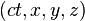$(ct, x, y, z)$

They can also be denoted by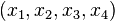$(x_1, x_2, x_3, x_4)$

Where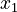$x_1$ represents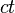$ct$. The reason for measuring time in units of the speed of light times the time coordinate is so that the units for time are the same as the units for space. Spacetime has the differential for arc length given by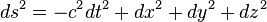$ds^2=-c^2dt^2+dx^2+dy^2+dz^2$

This implies that spacetime has a metric tensor given by$g_{uv}=\begin{bmatrix}-1&0&0&0\\0&1&0&0\\0&0&1&0\\0&0&0&1\end{bmatrix}$

As before stated, spacetime is flat everywhere; to some extent, it can be thought of as a plane.

### Simple

Spacetime can be thought of as the "arena" in which all of the events in the universe take place. All that one needs to specify a point in spacetime is a certain time and a typical spatial orientation. It is hard (virtually impossible) to visualize four dimensions, but some analogy can be made, using the method below.

## Spacetime diagrams

Hermann Minkowski introduced a certain method for graphing coordinate systems in Minkowski spacetime. As seen to the right, different coordinate systems will disagree on an object's spatial orientation and/or position in time. As you can see from the diagram, there is only one spatial axis (the x-axis) and one time axis (the ct-axis). If need be, one can introduce an extra spatial dimension, (the y-axis); unfortunately, this is the limit to the number of dimensions: graphing in four dimensions is impossible. The rule for graphing in Minkowski spacetime goes as follows:

1) The angle between the x-axis and the x'-axis is given by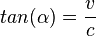$tan (\alpha)=\frac{v}{c}$ where v is the velocity of the object

2) The speed of light through spacetime always makes an angle of 45 degrees with either axis.

## Spacetime in general relativity

In the general theory of relativity, Einstein used the equation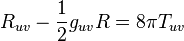$R_{uv}-\frac{1}{2}g_{uv}R=8 \pi T_{uv}$

To allow for spacetime to actually curve; the resulting effects are those of gravity.

## Images for kidsMinkowski spacetime Facts for Kids. Kiddle Encyclopedia.